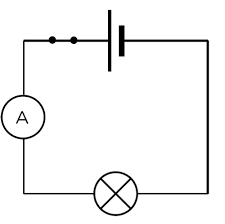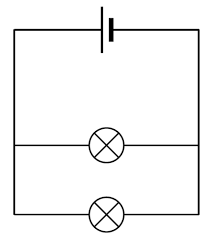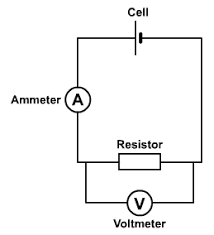# Electric Circuits

## Electrical Components

Warning: This lesson might include some technical words without definition. These will be covered in subsequent lessons in this topic, though feel free to do a cheeky google yourself first!

(LDR = Light dependent resistor)

(LED = Light emitting diode)

You need to be able to recognise and draw each and every one of these circuit symbols.

Each component has a different function:

• Switch (Open or Closed): Switches are used as an easy way to make or break a circuit, turning it on or off.
• Cell: Cells give power to the circuit, they store chemical energy. In everyday life, lots of people incorrectly call these batteries.
• Battery: Batteries are two or more cells in a circuit together.
• Diode: A diode only allows the current to flow one way. There are different types of diode, one of which we will meet later on.
• Resistor: A resistor provides a constant resistance to the circuit. This is important if you want to have a specific current or potential difference running through other components in the circuit.
• Variable Resistor: A resistor where you can change its resistance. They often have a dial to allow the resistance to be changed.
• LED: This stands for a light emitting diode. Remember what a diode does? This is a special kind of diode which releases light. LEDs are often used in torches and lights in a home.
• Lamp: Also known as a bulb and releases light when energy passes through it.
• Fuse: A fuse is a safety component. If the current becomes to high, it melts and breaks the circuit preventing a fire or damage. This is discussed in more detail in a further lesson.
• Voltmeter: Used to measure potential difference, this is always placed in parallel
• Ammeter: Used to measure current, this is always placed in series.
• Thermistor: A resistor changes its resistance according to its temperature. This is discussed in more detail in a further lesson.
• LDR: Light dependent resistor. This resistor changes its resistance according to how much light is shone onto it. This is discussed in more detail in a further lesson.
• Motor: Converts the chemical energy from the circuit into kinetic energy

Drawing circuits is relatively simple so long as you follow the following general rules:

1. Read the instructions carefully!!! I cannot tell you how many times students do not read the question properly and get it wrong.
2. Reread rule 1!
3. Use a ruler
4. Always ensure that there is either a battery or a cell drawn correctly.
5. If an ammeter is required, always draw it as part of the same loop as the battery/cell.
6. If a voltmeter is required, draw it around the component that you need to measure e.g. bulb or resistor

## Series and Parallel

A series circuit is a circuit with only one loop:A parallel circuit is a circuit with multiple loops:## Voltmeters

Potential difference is the energy transferred per unit charge. This means that if we have a battery of 5V, it gives every electron 5J of energy.

Voltmeters are always connected in parallel around the component as shown below.## Energy

You need to be able to recall and use the following equation:

E = Q x V

• E - Energy (Joules, J)
• Q - Charge (Coulombs, C)
• V - Potential Difference (Volts, V)
What is the difference between a series and parallel circuit?
Your answer should include: one loop / multiple loops
Explanation: Series only has one loop Parallel has multiple/more than one loop
A voltmeter is placed around a bulb and it measure 10V. How much energy is each electron giving to the bulb?
10J
Calculate the charge of a battery that gives 30J of energy to the circuit and has a potential difference of 25V
1.2C